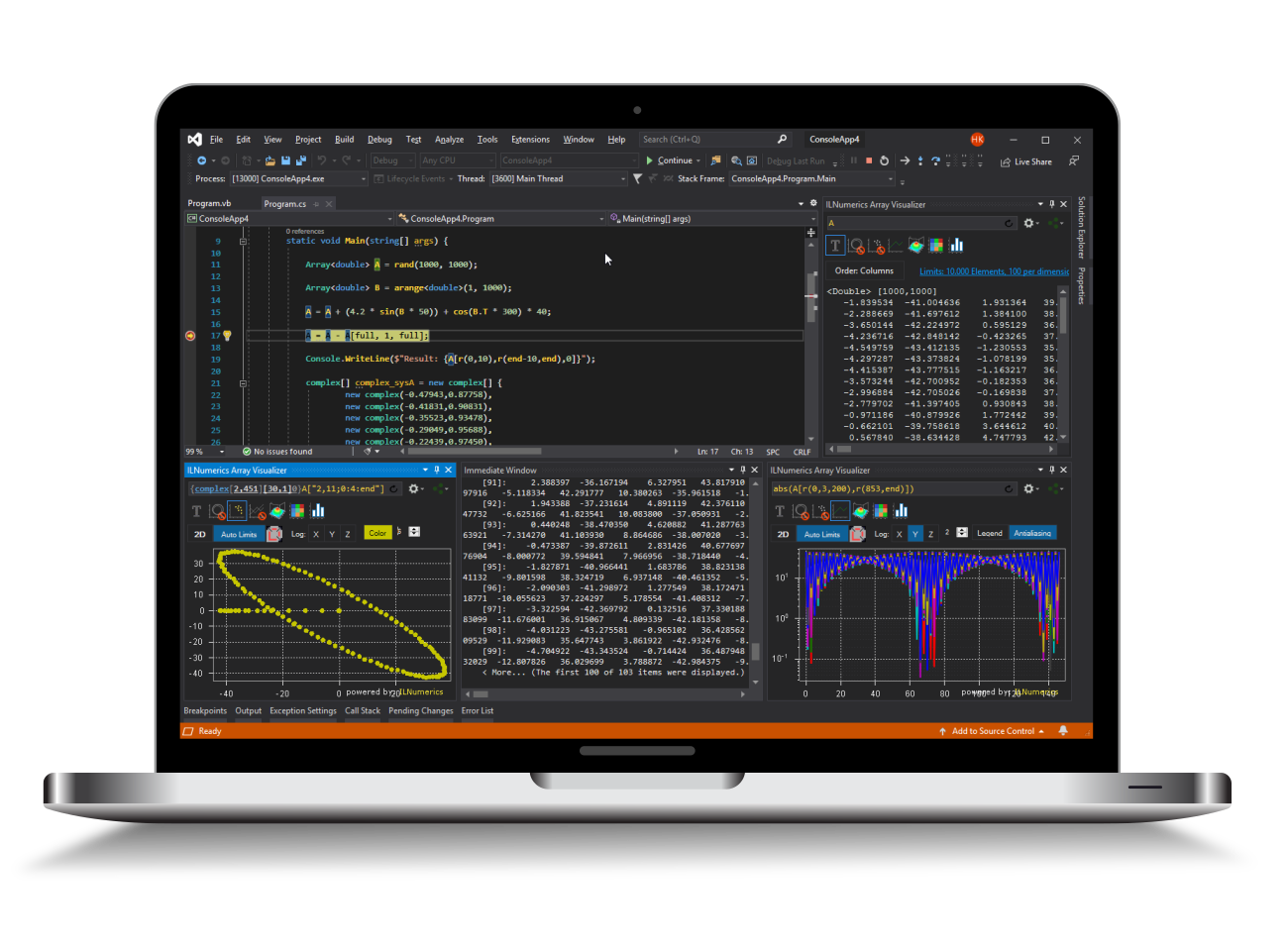Industrial Data Science
in C# and .NET:
Simple. Fast. Reliable.ILNumerics - Technical Computing

Modern High Performance Tools for Technical

Computing and Visualization in Industry and Science

tgt

# Pseudo inverse of a matrix in .NET (C#, Visual Basic)

The pseudo inverse of a matrix is a generalization of the inverse of a matrix for general matrices $A$. $A$ does not need to be square or non-singular. The pseudo inverse of $A$ is called $A^+$ and has some properties similar to the inverse of a matrix.

$$A \cdot A^+ \cdot A = A$$

$$A^+ \cdot A \cdot A^+ = A^+$$

The above equations do not imply that $A \cdot A^+$ is equal to the identity matrix.

## Calculating the pseudo inverse of a matrix in .NET (C#, Visual Basic)

The pseudo inverse is calculated by using the SVD of $A$ and then inverting the singular values. The tolerance for the inversion can be set explicitly.